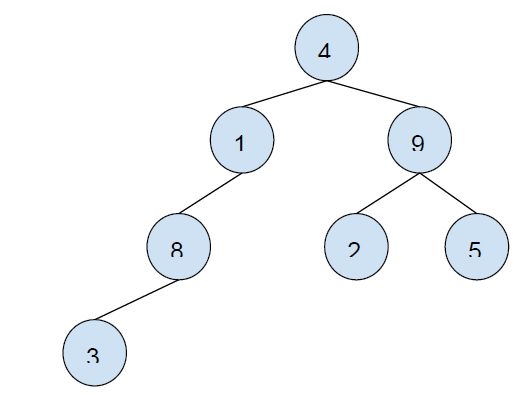# Binary tree to string with brackets in C++

In this problem, we are given a binary tree. Our task is to create a program that will convert a binary tree to string with brackets in C++.

The values of the binary tree are integers and it will be fed to the program in a preorder traversing way. The string should contain only integers and parentheses (), also it should be optimized i.e. all the empty pairs should be eliminated.

Binary Tree is a tree that has a special condition that each node can have a maximum of two children.

## Example of a binary tree −Preorder traversal : [4, 1, 8, 3, 9, 2, 5]

Let’s take an example to understand the problem

## Input

preorder: [4, 1, 8, 3, 9, 2, 5]## Output

### Explanation

Root -> 4()() -> 4(1()())(9) -> 4(1(8()())())(9) -> 4(1(8(3)())())(9) -> 4(1(8(3)())())(9(2)(5))

Eliminating all empty parentheses,

4(1(8(3)))(9(2)(5))

Now, let’s solve the problem, we will do the preorder traversal of the binary tree and we will place brackets wherever necessary. Also, we will have to eliminate the extra pair of braces. For this, we will use recursive calling to a function that will place brackets.

we will print a node and call the recursive function for both the child of the node with sentences till there are no child left for a node i.e. leaf node.

While calling the function for a node’s child nodes we will encounter one of these 4 cases −

Case1 − When the node has both child nodes present. We will place brackets for both child and place their values inside the brackets and call their child nodes if any.

Example − from the example above for the root node, 4 where both child nodes are present, 4(1)(9).

Case 2 − When node has left-child only. We will put the left child in the bracket, as no right child is present its bracket will be eliminated. And only left child’s subtrees are called if any.

Example − from the example above for node with value 1 where only the left child node is present, 4(1(8()()))(9).

Case 3 − When the node has the right-child only. We will put an empty bracket for the left child. And the value of the right child will be placed and its sub-trees will be called if any.

Case 4 − When the node has no child (leaf node). We will not put any brackets and just the value.

Example − from the example above for nodes with value 5 where no child is present, 4(1(8(3)))(9(2)(5()())).

## Program to convert binary tree to strings with brackets

//Program to convert binary tree to strings with brackets

## Example

Live Demo

#include <iostream>
using namespace std;
struct Node {
int data;
Node *left, *right;
};
Node* insertNode(int data){
Node* node = (Node*)malloc(sizeof(Node));
node->data = data;
node->left = node->right = NULL;
return (node);
}
void ConveryBinaryTreeToString(Node* root, string& str){
if (root == NULL)
return;
str.push_back(root->data + '0');
if (!root->left && !root->right)
return;
str.push_back('(');
ConveryBinaryTreeToString(root->left, str);
str.push_back(')');
if (root->right) {
str.push_back('(');
ConveryBinaryTreeToString(root->right, str);
str.push_back(')');
}
}
int main() {
struct Node* root = insertNode(4);
root->left = insertNode(1);
root->right = insertNode(9);
root->left->left = insertNode(8);
root->left->left->left = insertNode(3);
root->right->left = insertNode(2);
root->right->right = insertNode(5);
string binaryTreeString = "";
ConveryBinaryTreeToString(root, binaryTreeString);
cout<<"The string with preorder traversal of binary tree with brackets is: "<<binaryTreeString;
}

## Output

The string with preorder traversal of binary tree with brackets is: 4(1(8(3)))(9(2)(5))